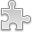# Courses / Module

Toggle Print

##LINEAR MODELS

Module code: ST303
Credits: 5
Semester: 1
Department: MATHEMATICS AND STATISTICS
International:Overview

Scatterplots and regression. The multiple regression model and its applications. Matrix notation, mean vectors and covariance matrices. Least-squares estimation. The multivariate normal distribution. Hypothesis testing and confidence intervals, prediction. Simple linear regression as a special case. Analysis of variance, R2, sequential sums of squares, general F-tests. Added variable plots. Model checking via testing for lack of fit and residual plots. Regression diagnostics: residuals, leverage, outliers, influence and Cook's Distance. Polynomials and factors in regression. Weighted least squares.Learning OutcomesTeaching & Learning methodsAssessmentAutumn Supplementals/ResitsPre-RequisitesTimetable